This tutorial will introduce you to the concept of a range in Google Sheets. You'll learn about what ranges are and how to use them. You'll also learn about named ranges and the benefits of using them.

A range represents a single cell or a group of adjacent cells in your spreadsheet. Every time you work with data in a spreadsheet, you're likely using one or more ranges.

The screenshot below shows 5 different ranges in Sheet3 of your spreadsheet.To reference a single cell in a formula, use the name of the sheet followed by an exclamation mark, the column and finally the row.

A cell that is in Sheet1 at the intersection of column C and row 5 will have the following reference:

. This type of reference is known as A1 notation.To reference a range composed of a group of adjacent cells, we'll need to specify the two cells that are at corners of any diagonal within the range. Typically, the cells that are at the top left and bottom right corner are the ones that are specified.

🛈 If the group of cells is fully contained within a single row or column then the top left and bottom right cells are just the first and last cells in the group.

The screenshot below displays multiple ranges (the ones that have been colored) and in each case the top left and bottom right cells have been filled with a darker color.To reference a group of cells in a formula, use the name of the sheet followed by an exclamation mark, the column of the top left cell, its row, a colon, the row of the bottom right cell and finally its column.

For example, the below references correspond to the ranges highlighted in the above screenshot.

• Range colored green:

• Range colored blue:

• Range colored: purple:

• Range colored orange:

You can also define ranges that reference entire rows or columns:

• All rows in one column: (use the column name twice and omit the row numbers)

• All rows in multiple adjacent columns: (use the names of the first and last column in the range and omit the row numbers)

• All columns in a single row: (use the row number twice and omit the column names)

• All columns in multiple adjacent rows: (use the numbers of the first and last row in the range and omit the column names)

To use a range in a function, just use the range's reference. For example, in order to calculate the sum of values in the range , use the formula . To sum values in just a single cell, say , use .In Google Sheets, you can assign a name to a range. Once you do this, you can use the name of a range instead of its reference in formulas and scripts.

There are several ways to create a named range:

1. Select Data —> Named ranges and enter the name and reference.

2. Select a range in the spreadsheet, right click and select Define named range to give it a name.

3. Create a named range by using Google Apps Script.

You can also create named ranges using Google Apps Script. The code below shows you an example of how to do that.

function createNamedRange(name, range) { SpreadsheetApp.getActive().setNamedRange(name, range); }

Once you create a named range, you can use it in formulas and scripts by using its name. So, instead of , you can use the range's name like this: .

Using named ranges has several benefits:

• Formulas and scripts will become more readable because the name of the range will help users understand the type of data contained in it. For e.g., is a lot more descriptive than .

• When you make changes to a named range, all the formulas and scripts will immediately begin using the updated range.

This tutorial covered the basics of working with ranges in Google Sheets. You learned how to:

Have feedback for me?

I'd appreciate any feedback you can give me regarding this post.

Was it useful? Are there any errors or was something confusing? Would you like me to write a post about a related topic? Any other feedback is also welcome. Thank you so much!

## How to Create Named Ranges in Google Sheets (Static & Dynamic)

ShareShareTweetEmailPrint

Named Ranges in Google Sheets can be used to define a cell or a range of cells and give it a name. Now, instead of using the cell references, you can use the name of the named range in calculations.

For example, suppose I have sales transaction data for a store, and I need to calculate metrics such as the sum of all the sales, count of sales of item A, average sale value, maximum/minimum sale value.

Instead of using the cell references of the range that has the sales data, I can create a named range (with a descriptive name such as ‘SalesData’) and use this name instead.

Using Named Ranges have the following benefits:

1. Named Ranges are easier to use in formulas. You can give a descriptive name to named ranges, and you don’t have to worry about knowing the range reference. For example, =AVERAGE(SalesData) is easier than =AVERAGE(B2:B21)
2. You can update a named range and all the formulas using it would now refer to the new range. This is a big time saver.

### Creating a Named Range in Google Sheets

Suppose I have a sales transaction data as shown below, and I want to create a named range for the sales data: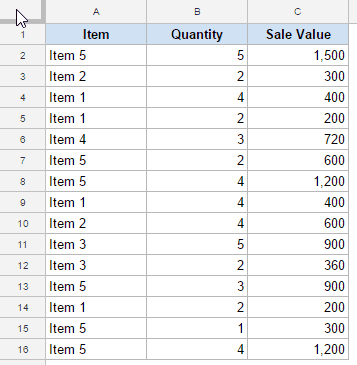Here are the steps to create a named range in Google Sheets:

• Select the data for which you want to create a named range.
• Go to the Data Tab.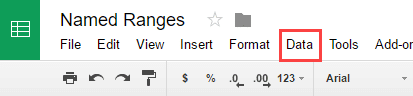• Click on Named Ranges from the drop down.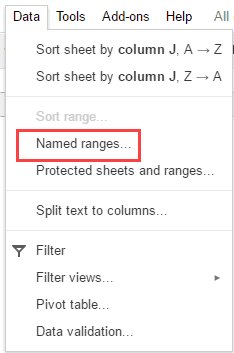• In the Named ranges pane, enter the name in the first field box. In this example, we are using the name SalesData.
• Make sure the right data range is listed in the second field box. If incorrect, change it.
• Click on Done.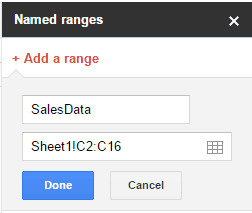This will create the Named Range – SalesData.

Once created, you can use the named range in formulas. Here are some examples where you can use the SalesData named range:

• To get the sum of all the sales: =SUM(SalesData)
• To find the average sales value: =AVERAGE(SalesData)
• To find the maximum sales value: =MAX(SalesData)

### Naming Rules While Creating Named Ranges in Google Sheets

There are some rules you need to follow while creating named ranges in Google Sheets.

A named range:

• Can’t contain any spaces or punctuation. For example, you can not have ‘Sales Data’. However, you can use underscore between words. Hence ‘Sales_Data’ is fine.
• Can contain only letters, numbers, and underscores.
• Can’t start with a number, or the words “true” or “false.”
• Must not be more than 250 characters long.
• Can’t be something that refers to a range. For example, you can not name it A1, as it already refers to a cell. Similarly, you can not name it A1:A100.

### Editing a Named Range

Once a Named Range is created, you may want to change it or update it.

For example, if you have a named range that refers to the sales data, and you have three new transactions that need to be added to the data, you would have to update the named range to include these three additional data points.

Here are the steps to update/edit a named range in Google Sheets:

• Go to the Data Tab.
• Click on Named Ranges.
• Hover the mouse on the Named range that you want to edit and click on the Edit icon.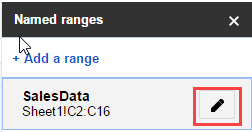• Update the Name or the Range.
• Click on Done.

### Creating a Dynamic Named Range in Google Sheets

Named Ranges are great as it allows you to update the named range once and all the formulas using that named range automatically update.

However, doing this manually may seem tedious if you have to frequently update the data or if you have too many named ranges.

While in Excel, it’s possible to create a named range using the OFFSET or INDIRECT formula, it doesn’t work in Google Sheets.

However, you can do that using a neat INDIRECT function trick.

Suppose you have a dataset as shown below and you want to create a named range for the sales data in a way that whenever new data is added, the named range automatically updates.

Here are the steps to create a dynamic named range in Google Sheets:

• In a cell (E2 in this example) enter the formula =COUNT(C2:C100)+1. This will give us the number of cells that have a number in it. 1 is added to the formula as our sales data starts from row number 2. Also note that we have used C2:C100 so that if data is added in future, it will automatically be counted. Also, we have used COUNT function as the data is all numeric. Based on your data, you can use COUNTIF as well.• In another cell (F2 in this example), enter the formula =“Sheet1!C2:C”&E2. This would give us a reference that would cover the entire sales data column. For example, if there are 10 sales transactions, it would give Sheet1!C2:C11. If there are 15 transactions, it will give Sheet1!C2:C16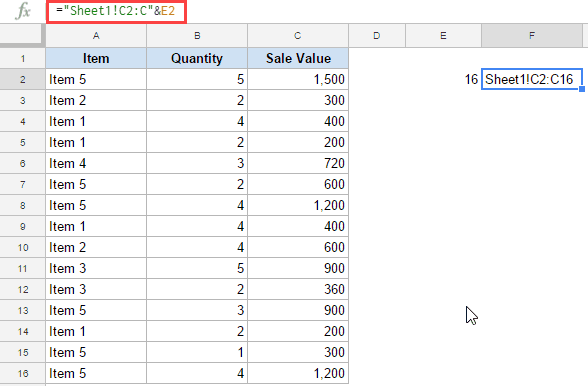• Go to the Data tab and select Named Ranges.
• Create a named range with the name SalesData and use Sheet1!F2 as the range.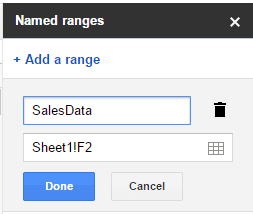Now, you can use the following formula to refer to the dynamic named range: =INDIRECT(SalesData).

The INDIRECT function would use the named range and refer to cell F2, which in turn has the reference for the sales data. Since we have made the range in F2 dynamic (by using =“Sheet1!C2:C”&E2), the named range also becomes dynamic.

For example,  if you now want to calculate the sum of sales, you can use the formula =SUM(INDIRECT(SalesData)). If more transaction records are added, the formula would automatically update and give you the new sum of sales.

You May Also Like the Following Google Sheets Tutorials:##### Sumit

Google Sheets and Microsoft Excel Expert.

When handling large amounts of data, being able to group certain values together can be very useful. Calculating hundreds of values automatically is one of the reasons that spreadsheets were made, after all. This is where being able to declare cell ranges is important, as it simplifies what would be otherwise cumbersome computations.### How to Find the Range in Google Sheets

The definition of a range in spreadsheets is quite different from its equivalent in math. Simply put, when working in spreadsheet programs, a range is a group of selected cells. This is important because by grouping cells together, you can use these groups as values for doing calculations. This allows a user to automatically compute formulas with a range as an argument.

Finding the range in Google Sheets is a very easy process. You just start from one end of a data set to the other. For example, a data set of ten numbers has a range either from one to ten or from ten to one. It doesn’t matter where you begin or where you end, as long as it covers the entire data set, that is your range.

If you look at the top and to the left of a Google Sheet document, you’ll notice that some letters and numbers mark them. This is how you determine the name of a particular cell in the sheet. You look at the letter from the top, then look at the number to the left. The very first cell would be A1, the cell immediately to the bottom of it would be A2, and the one to the immediate right is B2. This is how you determine the first and last value of your range.

Calculating the range would be easy if it was a single row or a column. Just use both ends of the data set that has a value then put a colon in between them. For example, in one column of data starting from A1 to A10, the range would be A1:A10 or A10:A1. It doesn’t matter if you use either end first.

It gets a little complicated when you’re working with multiple rows or columns. For this kind of data set, you need to determine two opposite corners to get your range. For example, a set of nine cells composed of three rows and three columns starting from A1 and ending at C3, the opposite corners would be A1 and C3 or A3 and C1.

It makes no difference whether you take the top leftmost and the bottom rightmost cells or the bottom leftmost and the top rightmost. As long as they’re opposite corners, you will cover the entire data set. The range would then be either A1:C3, C3:A1, A3:C1, or C1:A3. It doesn’t matter which cell you use as your first range value.

Finding the value of a range by typing in values is handy when the number of data values you have is too many to be able to select it manually. Otherwise, you can type in = in an empty cell, then click and drag your mouse over the entire data set to automatically generate a data range.

### How to Create Named Ranges in Google Sheets

Named ranges become useful when you have too many range sets to keep track of. This can also help simplify calculations, as you can use the labels themselves as arguments for formulas. What’s easier to remember? =sum(a1:a10) or =sum(daily_sales)? By using the latter, not only will you know what the range is actually for, by looking at the formula alone you can see that the result is the sum of the day’s sales.

To create a named range, do the following:2. Select the range you want to name.3. Click on Data on the top menu.4. Click on Named ranges from the dropdown list. A window will pop up on the right.5. On the first textbox, type in the name that you want.6. If you wish to change the selected range, you can change the values on the second textbox. If you have multiple sheets, you can type the sheet name followed by an exclamation mark (!) to specify which sheet you’re using. The values between colon (:) is the range.7. Once you’re finished naming, click on Done.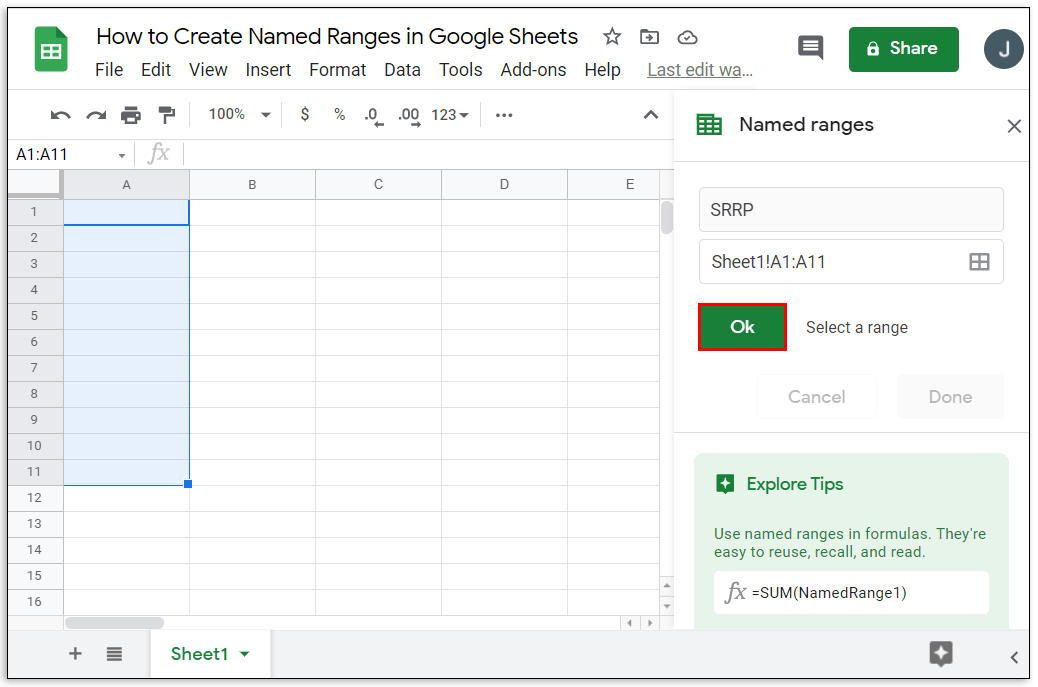There are certain rules that you must follow when naming ranges. Not adhering to these rules will often result in error messages or failure of a formula to produce a result. These rules are:

1. Range names can only contain numbers, letters, and underscores.
2. You can’t use spaces or punctuation marks.
3. Range names can’t begin with either the word true or false.
4. The name must be between one and 250 characters.

Here’s how to edit already named ranges:2. Click on Data on the top menu.3. Click on Named ranges from the dropdown menu.4. On the window to the right, click on the named range that you want to edit.5. Click on the pencil icon to the right.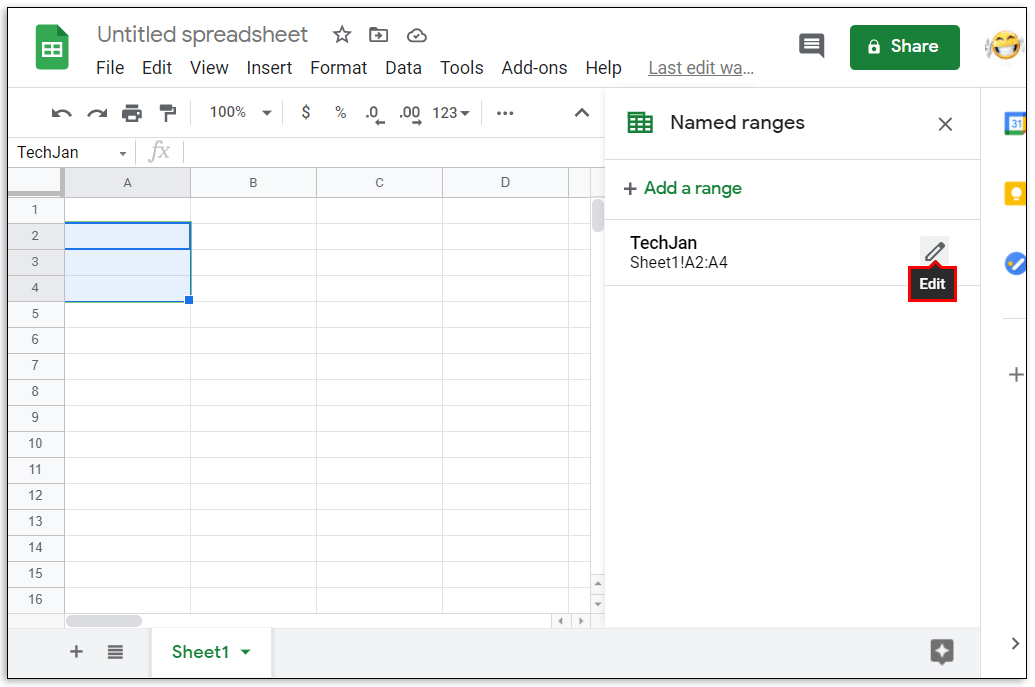6. To edit the name, type in the new name then click on Done. To delete the range name, click on the trash can icon to the right of the range name, then click on Remove on the window that pops up.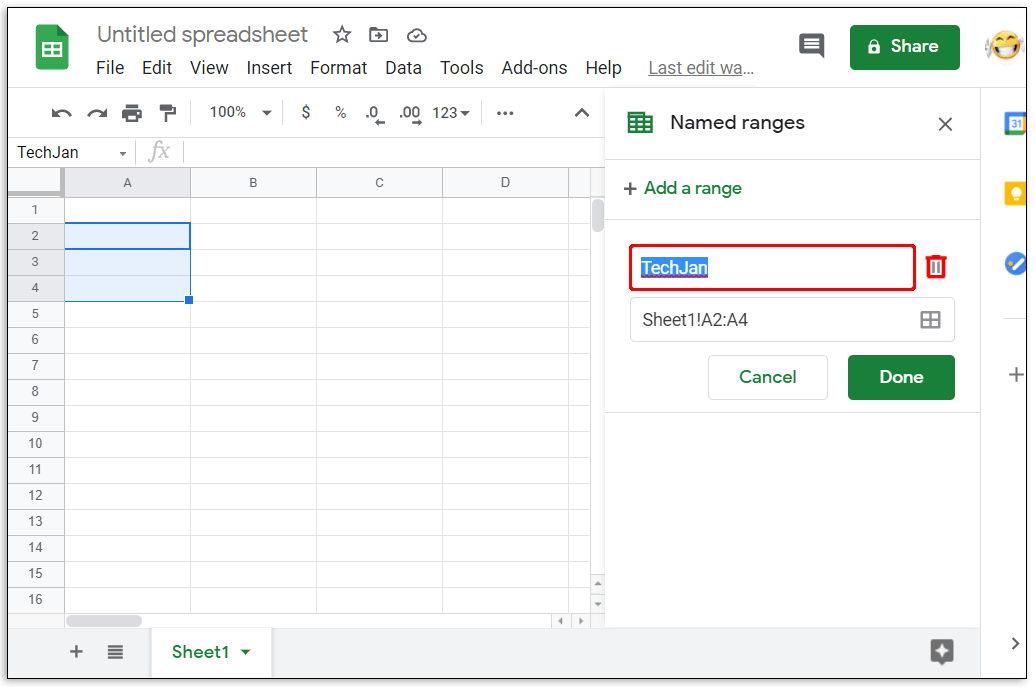### How Do You Access AVERAGE Function in Google Sheets?

If you want to use the AVERAGE function, you can do the following:

• Click on an empty cell where you wish the answer to be displayed.• On the top menu, click on Insert.• Mouse over Function on the dropdown menu.• Click on AVERAGE.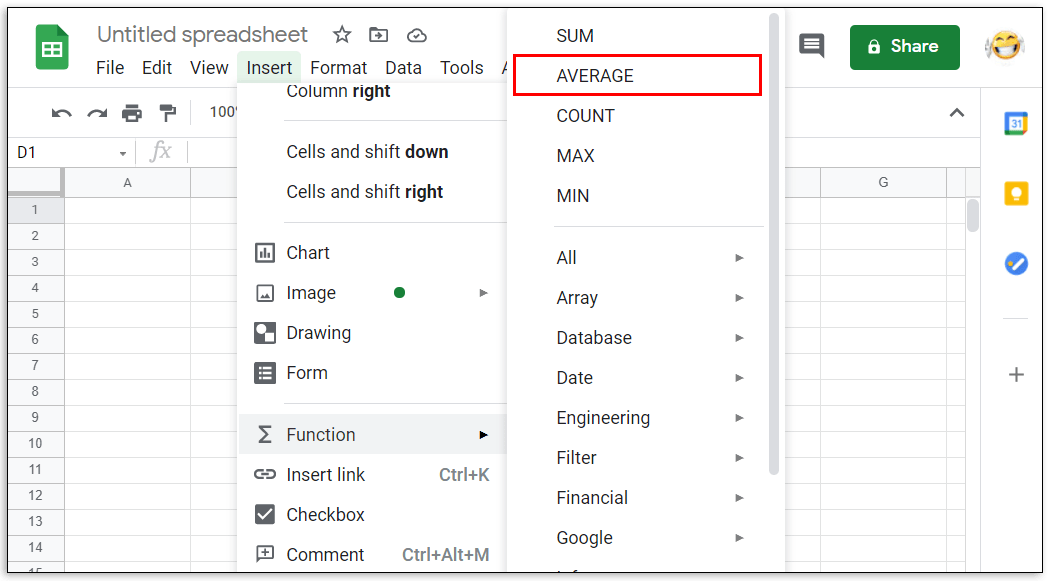• Type in the values that you want the AVERAGE function to use.• Press the enter or return key.

Changing the range is as easy as editing the first or last value of the cell numbers between the colon symbol. Remember that the range argument takes the first and last value you enter and includes all cells in between as a member of that range. Increasing or decreasing either number in between the colon will increase or decrease the members of the range accordingly.

### How Do You Calculate Total in Google Sheets?

Formulas in Google Sheets can automatically calculate the total of a certain range of cells. If the values inside the cells are changed, the total will then adjust accordingly. The usual function used is SUM which is the total of all the values in the argument. The syntax of this function is =SUM(x:y) where x and y is the start and end of your range accordingly. For example, the total of a range from A1 to C3 will be written as =SUM(A1:C3).

### How Do I Select a Data Range in Google Sheets?

You can select a range in two ways, either type in the range values manually, or click and drag your mouse over the entire range itself. Clicking and dragging is useful if the amount of data you have only spans a few pages. This becomes unwieldy if you have data that number in the thousands.

To manually select a data range, find the top leftmost value and the bottom rightmost value and place them in between a colon. The same also applies to the top rightmost and bottom leftmost values. You can then type this in as an argument in a function.

### How Do You Find the Mean in Google Sheets?

In mathematical terms, the mean is the sum of the values of a set of cells, divided by the number of cells added. Simply put, it is the average value of all the cells. This can be accomplished by using the AVERAGE function in the Insert and Function menu.

### What Is a Data Range in Google Sheets?

A data range is a set of cells that you want to use in a function or formula. It’s another name for range. The two names are interchangeable.

### What Is a Valid Range in Google Sheets?

Depending on the formula you use, some values will not be accepted as an argument. For example, the cell value TRUE can’t be used in the formula =SUM() as it’s not a calculable numeric value. A valid range is a set of cells containing data that a formula will accept as an argument. If there is a cell that has an unaccepted input, then the range isn’t valid. Invalid ranges can also occur when either the first or last point of the range has a value that results in an error.

### How Do I Find the Statistical Range of Values in Google Sheets?

In mathematics, the statistical range is the difference between the highest value and the lowest value of a set of data. Google Sheets has several functions that make the calculation of this rather simple. The MAX and MIN function is located under the Insert and Function menu. To find the statistical range or a data set just type in =(MAX(x) – MIN(x)) where x is your range. For the statistical range of a data set from A1 to A10, for example, the formula would be =(MAX(A1:A10) – MIN(A1:A10)). If you want rounded down values, you can use this syntax: =round(MAX(A1:A10),1)-round(MIN(A1:A10),1).

### Efficient Calculations

Knowing how to calculate the range in Google Sheets helps users efficiently handle vast amounts of data. You can make use of all the formulas and functions that Google Sheets has to offer more easily if you can group data in specific sets and ranges. Understanding how ranges work can help simplify your workload.

Do you know of another way on how to calculate a range in Google Sheets? Share your thoughts in the comments section below.

Dynamic Named Ranges in Google Sheets (20121)

Debt is sacred, Jane croaked, laughing, which made him a little taken aback. - Look, she also laughs. The woman has no brains at all.

Nausea rose in my throat. After waiting for me to catch my breath, my mother ordered me to stand near the curtain that closed the balcony door, and she herself stood with a. Camera at the other end of the room. I got out of bed and trudged to the indicated place.

Google Sheets - Named Ranges, Dynamic Updates, with IMPORTRANGE, Other Sheets, Arrays - Tutorial

No more. Four. Okay.

### Now discussing:

You are my beloved angel, relax, give your uncle what he wants, and I will be very kind to you, he repeated, squeezing my chest. Then he put me on my side, continuing to have in the ass. Kolyan waited until I finished arching and continued to push his penis into my anus. He entered heavily, with extraordinary friction.

Having driven him completely, he paused and backed away.

2275 2276 2277 2278 2279